# CLASS 12 MATHS CHAPTER 9-DIFFERENTIAL EQUATIONS

### Solve The Following Questions.

Determine order and degree (if defined) of differential equations given in Questions 1 to 10:

Question1.

Solution :
Given:

The highest order derivative present in the differential equation is y”” and its order is 4.

The given differential equation is not a polynomial equation in its derivatives. Hence, its degree is not defined.

Question2. y’ + 5y = 0

Solution :

The given differential equation is:

y’ + 5y = 0

The highest order derivative present in the differential equation is y’. Therefore, its order is one.

It is a polynomial equation in y’. The highest power raised to y’is 1. Hence, its degree is one.

Question3.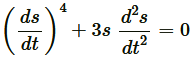Solution :
Given:The highest order derivative present in the given differential equation is d2s/dt2 Therefore, its order is two.

It is a polynomial equation in d2s/dtand ds/dt. The power raised to d2s/dt2 is 1.

Hence, its degree is one.

Question4.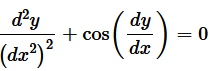Solution :
Given:The highest order derivative present in the given differential equation is d2y/dx Therefore, its order is 2.

The given differential equation is not a polynomial equation in its derivatives. Hence, its degree is not defined.

Question5.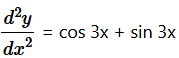Solution :
Given:The highest order derivative present in the differential equation is d2y/dx2  and its order is 2.

The given differential equation is a polynomial equation in derivatives and the highest power raised to highest order d2y/dx2  is one, so its degree is 1.

Hence, order is 2 and degree is 1.

Question6.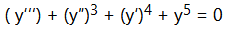Solution :
Given:The highest order derivative present in the differential equation is y”’. Therefore, its order is three.

The given differential equation is a polynomial equation in y”’, y”, and y’.

The highest power raised to y”’ is 2. Hence, its degree is 2.

Question7. y”’ + 2y” + y’ = 0

Solution :
Given: y”’ + 2y” + y’ = 0

The highest order derivative present in the differential equation is y”’. Therefore, its order is three.

It is a polynomial equation in y”’, y” and y’. The highest power raised to y”’is 1. Hence, its degree is 1.

Question8. y′ + y = ex

Solution :
Given: y′ + y = ex

⇒ y′ + y – ex = 0

The highest order derivative present in the differential equation is y’. Therefore, its order is one.

The given differential equation is a polynomial equation in y’ and the highest power raised to y’ is one. Hence, its degree is one.

Question9. y’′ + (y’)2 + 2y = 0

Solution :
Given: y’′ + (y’)2 + 2y = 0

The highest order derivative present in the differential equation is y”. Therefore, its order is two.

The given differential equation is a polynomial equation in y”and y’ and the highest power raised to y” is one.

Hence, its degree is one.

Question10. y’′ + 2y’ + sin y = 0

Solution :
Given: y’′ + 2y’ + sin y = 0

The highest order derivative present in the differential equation is y”. Therefore, its order is two.

This is a polynomial equation in y”. and y’.and the highest power raised to y”. is one. Hence, its degree is one.

Question11. The degree of the differential equation  is:

(A) 3

(B) 2

(C) 1

(D) Not defined

Solution :
Given:  ……….(i)

The given differential equation is not a polynomial equation in its derivatives. Therefore, its degree is not defined.

Hence, the correct answer is D.

Hence, option (D) is correct.

Question12. The order of the differential equation  is:

(A) 2

(B) 1

(C) 0

(D) Not defined

Solution :
Given:

The highest order derivative present in the differential equation is d2y/dx2  and its order is 2.

Therefore, option (A) is correct.

### Solve The Following Questions.

In each of the Questions 1 to 6 verify that the given functions (explicit) is a solution of the corresponding differential equation:

Question1.  y = ex  + 1  :  y″ – y′ = 0

Solution :
Given: y = ex  + 1

Thus, the given function is the solution of the corresponding differential equation.

Question2.  y = x2 + 2x + C  :  y′ – 2x – 2 = 0

Solution :
Given: y = x2 + 2x + C

Differentiating both sides of this equation with respect to x, we get:

Hence, the given function is the solution of the corresponding differential equation.

Question3. y = cos x + C : y′ + sin x = 0

Solution :
Given: y = cos x + C

Differentiating both sides of this equation with respect to x, we get:

Hence, the given function is the solution of the corresponding differential equation.

Question4.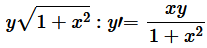Solution :
Given: y = √1 + x2

Hence, the given function is the solution of the corresponding differential equation.

Question5. y = Ax   :  xy′ = y (x ≠ 0)

Solution :
Given: y = Ax

Differentiating both sides with respect to x, we get:

Hence, the given function is the solution of the corresponding differential equation.

Question6.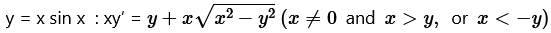Solution :
Given: y = x sin x

Differentiating both sides of this equation with respect to x, we get:

Hence, the given function is the solution of the corresponding differential equation.

In each of the questions 7 to 10, verify that the given functions (explicit or implicit) is a solution of the corresponding differential equation:

Question7. xy = log y + C :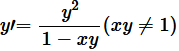Solution :
Given: xy = log y + C

Differentiating both sides of this equation with respect to x, we get:

Hence, the given function is the solution of the corresponding differential equation.

Question8. y – cos y = x :  (y sin y + cos y + x) y′ = y

Solution :
Given: y – cos y = x

Differentiating both sides of the equation with respect to x, we get:

Hence, the given function is the solution of the corresponding differential equation.

Question9. x + y = tan-1 y   :   y2y’ + y+ 1 = 0

Solution :
Given: x + y = tan-1 y

Differentiating both sides of this equation with respect to x, we get:

Hence, the given function is the solution of the corresponding differential equation.

Question10.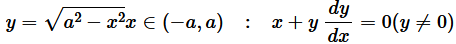Solution :
Given: y = √a2 – x2

Differentiating both sides of this equation with respect to x, we get:

Hence, the given function is the solution of the corresponding differential equation.

Question11. The number of arbitrary constants in the general solution of a differential equation of fourth order are:

(A) 0

(B) 2

(C) 3

(D) 4

Solution :
Option (D) is correct.

We know that the number of constants in the general solution of a differential equation of order n is equal to its order.

Therefore, the number of constants in the general equation of fourth order differential equation is four.

Question12. The number of arbitrary constants in the particular solution of a differential equation of third order are:

(A) 3

(B) 2

(C) 1

(D) 0

Solution :
The number of arbitrary constants in a particular solution of a differential equation of any order is zero (0) as a particular solution is a solution which contains no arbitrary constant.

Therefore, option (D) is correct.

### Solve The Following Questions.

In each of the questions 1 to 5, form a differential equation representing the given family of curves by eliminating arbitrary constants a and b

Question 1.

Solution :
Given: Equation of the family of curves ….(i)

Differentiating both sides of the given equation with respect to x, we get:

Hence, the required differential equation of the given curve is y” = 0.

Question 2.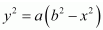Solution :
Given: Equation of the family of curvesDifferentiating both sides with respect to x, we get:

This is the required differential equation of the given curve.

Question 3.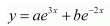Solution :
Given: Equation of the family of curves….(i)

Differentiating both sides with respect to x, we get:

This is the required differential equation of the given curve.

Question 4.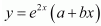Solution :
Given: Equation of the family of curvesDifferentiating both sides with respect to x, we get:

This is the required differential equation of the given curve.

Question 5.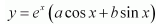Solution :
Given: Equation of the family of curves….(i)

Differentiating both sides with respect to x, we get:

This is the required differential equation of the given curve.

Question 6. Form the differential equation of the family of circles touching the y-axis at the origin.

Solution :
The centre of the circle touching the y-axis at origin lies on the x-axis.

Let (a, 0) be the centre of the circle.

Since it touches the y-axis at origin, its radius is a.

Now, the equation of the circle with centre (a, 0) and radius (a) is

This is the required differential equation.

Question 7. Find the differential equation of the family of parabolas having vertex at origin and axis along positive y-axis.

Solution :

The equation of the parabola having the vertex at origin and the axis along the positive y-axis is:

x2 = 4ay

Differentiating equation (1) with respect to x, we get:

2x = 4ay’

Dividing equation (2) by equation (1), we get:

This is the required differential equation.

Question 8. Form the differential equation of family of ellipse having foci on y-axis and centre at the origin.

Solution :

The equation of the family of ellipses having foci on the y-axis and the centre at origin is as follows:

This is the required differential equation.

Question 9. Form the differential equation of the family of hyperbolas having foci on x-axis and centre at the origin.

Solution :

The equation of the family of hyperbolas with the centre at origin and foci along the x-axis is: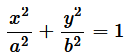….(i)

This is the required differential equation.

Question 10. Form the differential equation of the family of circles having centres on y-axis and radius 3 units.

Solution :

Let the centre of the circle on y-axis be (0, b).

The differential equation of the family of circles with centre at (0, b) and radius 3 is as follows:

This is the required differential equation.

Question 11. Which of the following differential equation has  as the general solution:

Solution :
Given: ….(i)

Differentiating with respect to x, we get:

This is the required differential equation of the given equation of curve.

Hence, the correct answer is B.

Question 12. Which of the following differential equations has y = x as one of its particular solutions:

Solution :

The given equation of curve is y = x.

Differentiating with respect to x, we get:

dy/dx = 1           …(1)

Again, differentiating with respect to x, we get:

d2y/dx= 0        ….(2)

Now, on substituting the values of y, d2y/dx2 and dy/dx  from equation (1) and (2) in each of the given alternatives, we find that only the differential equation given in alternative C is correct.

Therefore, option (C) is correct.

### Solve The Following Questions.

For each of the differential equations in Questions 1 to 4, find the general solution:

Question 1.

Solution :
Given: Differential equation

This is the required general solution of the given differential equation.

Question2.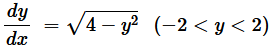Solution :
The given differential equation is:

This is the required general solution of the given differential equation.

Question 3.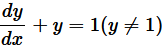Solution :
Given: Differential equation

This is the required general solution of the given differential equation.

Question4.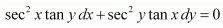Solution :
Given: Differential equation

This is the required general solution of the given differential equation.

Question 5.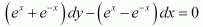Solution :
Given: Differential equationThis is the required general solution of the given differential equation.

Question 6.

Solution :
Given: Differential equation

Question 7. y log y dx – x dy = 0

Solution :
Given: Differential equation y log y dx – x dy = 0

This is the required general solution of the given differential equation.

Question 8.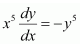Solution :
Given: Differential equationQuestion 9.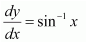Solution :
Given: Differential equationThis is the required general solution of the given differential equation.

Question 10.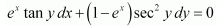Solution :
Given: Differential equationFor each of the differential equations in Question 11 to 12, find a particular solution satisfying the given condition:

Question 11.

Solution :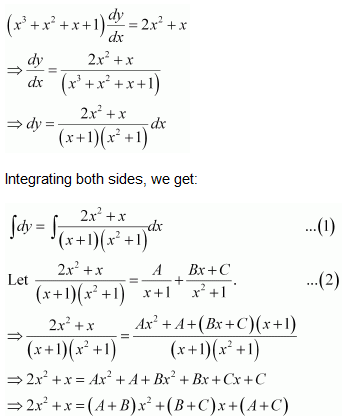Comparing the coefficients of x2 and x, we get:

A + B = 2

B + C = 1

A + = 0

Solving these equations, we get:

Question 12.

Solution :

For each of the differential equations in Question 13 to 14, find a particular solution satisfying the given condition.

Question 13.

Solution :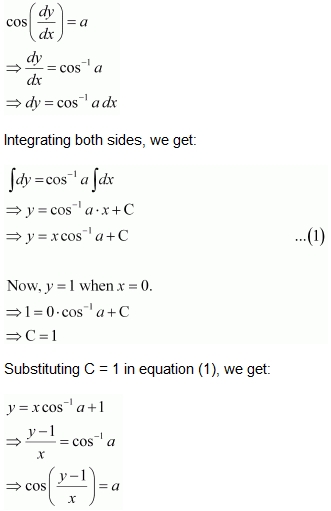Question 14.

Solution :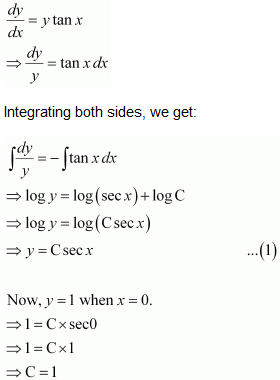Substituting C = 1 in equation (1), we get:

y = sec x

Question 15. Find the equation of the curve passing through the point (0, 0) and whose differential equation is y’ = ex sin x

Solution :

The differential equation of the curve is:

Question 16. For the differential equation find the solution curve passing through the point (1,-1)

Solution :

Question 17. Find the equation of the curve passing through the point (0,-2) given that at any point (x,y) on the curve the product of the slope of its tangent and y-coordinate of the point is equal to the x-coordinate of the point.

Solution :

Let and y be the x-coordinate and y-coordinate of the curve respectively.

We know that the slope of a tangent to the curve in the coordinate axis is given by the relation,

dy/dx

According to the given information, we get:

Now, the curve passes through point (0, –2).

∴ (–2)2 – 02 = 2C

⇒ 2C = 4

Substituting 2C = 4 in equation (1), we get:

y2 – x2 = 4

This is the required equation of the curve.

Question 18. At any point (xy) of a curve, the slope of the tangent is twice the slope of the line segment joining the point of contact to the point (–4, –3). Find the equation of the curve given that it passes through (–2, 1).

Solution :

It is given that (xy) is the point of contact of the curve and its tangent.

The slope (m1) of the line segment joining (xy) and (–4, –3) is y+3/x+4.

We know that the slope of the tangent to the curve is given by the relation,

dy/dx

Substituting C = 1 in equation (1), we get:

y + 3 = (x + 4)2

This is the required equation of the curve.

Question 19. The volume of the spherical balloon being inflated changes at a constant rate. If initially its radius is 3 units and after 3 seconds it is 6 units. Find the radius of balloon after t seconds.

Solution :

Let the rate of change of the volume of the balloon be k (where k is a constant).

⇒ 4π × 3= 3 (k × 0 + C)

⇒ 108π = 3C

⇒ C = 36π

At = 3, r = 6:

⇒ 4π × 63 = 3 (k × 3 + C)

⇒ 864π = 3 (3k + 36π)

⇒ 3k = –288π – 36π = 252π

⇒ k = 84π

Substituting the values of k and C in equation (1), we get:

Thus, the radius of the balloon after t seconds is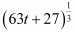.

Question 20. In a bank, principal increases continuously at the rate of r% per year. Find the value of r if Rs 100 doubles itself in 10 years (log­e 2 = 0.6931).

Solution :

Let pt, and r represent the principal, time, and rate of interest respectively.

It is given that the principal increases continuously at the rate of r% per year.

Hence, the value of r is 6.93%.

Question 21. In a bank, principal increases continuously at the rate of 5% per year. An amount of Rs 1000 is deposited with this bank, how much will it worth after 10 years

(e0.5 = 1.645).

Solution :

Let p and t be the principal and time respectively.

It is given that the principal increases continuously at the rate of 5% per year.

Hence, after 10 years the amount will worth Rs 1648.

Question 22. In a culture the bacteria count is 1,00,000. The number is increased by 10% in 2 hours. In how many hours will the count reach 2,00,000, if the rate of growth of bacteria is proportional to the number present.

Solution :

Let y be the number of bacteria at any instant t.

It is given that the rate of growth of the bacteria is proportional to the number present.

Question 23. The general solution of the differential equation is:

Solution :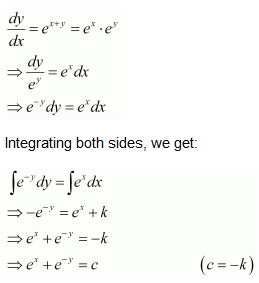Therefore, option (A) is correct.

### Solve The Following Questions.

In each of the following Questions 1 to 5, show that the differential equation is homogenous and solve each of them:

Question1.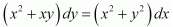Solution :
The given differential equation i.e., (x2 + xydy = (x2 + y2dx can be written as:

This shows that equation (1) is a homogeneous equation.

To solve it, we make the substitution as:

vx

Differentiating both sides with respect to x, we get:

dy/dx = v + x dv/dx

Substituting the values of v and dy/dx in equation (1), we get:

This is the required solution of the given differential equation.

Question2.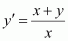Solution :

The given differential equation is:

Thus, the given equation is a homogeneous equation.

To solve it, we make the substitution as:

vx

Differentiating both sides with respect to x, we get:

dy/dx = v + x dv/dx

Substituting the values of y and dy/dx in equation (1), we get:

This is the required solution of the given differential equation.

Question3.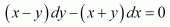Solution :

The given differential equation is

Question4.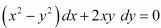Solution :

The given differential equation is:

Therefore, the given differential equation is a homogeneous equation.

To solve it, we make the substitution as:

vx

This is the required solution of the given differential equation.

Question5.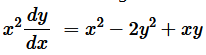Solution :

The given differential equation is:

Therefore, the given differential equation is a homogeneous equation.

To solve it, we make the substitution as:

vx

This is the required solution for the given differential equation.

In each of the Questions 6 to 10, show that the given differential equation is homogeneous and solve each of them:

Question6.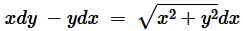Solution :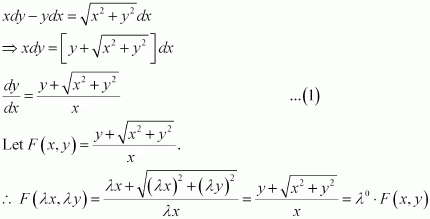Therefore, the given differential equation is a homogeneous equation.

To solve it, we make the substitution as:

vx

This is the required solution of the given differential equation.

Question7.

Solution :

The given differential equation is:

Therefore, the given differential equation is a homogeneous equation.

To solve it, we make the substitution as:

vx

This is the required solution of the given differential equation.

Question8.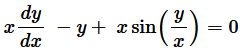Solution :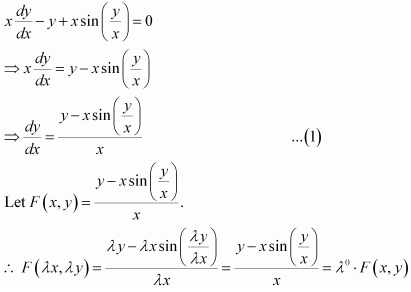Therefore, the given differential equation is a homogeneous equation.

To solve it, we make the substitution as:

vx

This is the required solution of the given differential equation.

Question9.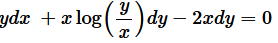Solution :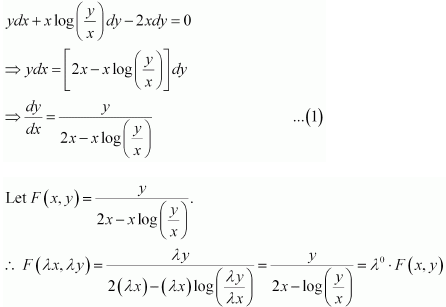Therefore, the given differential equation is a homogeneous equation.

To solve it, we make the substitution as: y = vx

Integrating both sides, we get:

This is the required solution of the given differential equation.

Question10.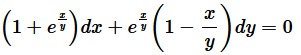Solution :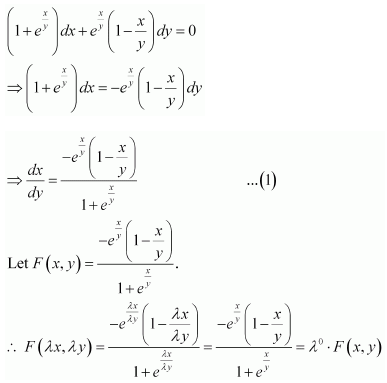Therefore, the given differential equation is a homogeneous equation.

To solve it, we make the substitution as: x = vy

This is the required solution of the given differential equation.

For each of the differential equations in Questions from 11 to 15, find the particular solution satisfying the given condition

Question11. (x + y) dy + (x – y) dx = 0; y = 1 when x = 1

Solution :

Therefore, the given differential equation is a homogeneous equation.

To solve it, we make the substitution as:

y = vx

Substituting the value of 2k in equation (2), we get:

This is the required solution of the given differential equation.

Question12.  x2 dy + (xy + y2 ) dx = 0; y = 1 when x = 1

Solution :

Therefore, the given differential equation is a homogeneous equation.

To solve it, we make the substitution as:

y = vx

This is the required solution of the given differential equation.

Question13.

Solution :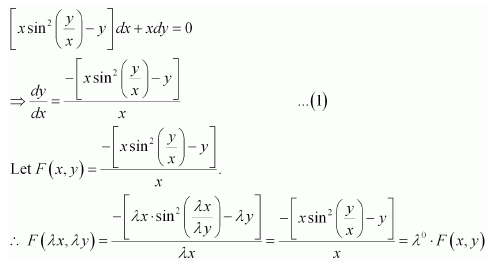Therefore, the given differential equation is a homogeneous equation.

To solve it, we make the substitution as:

y = vx

This is the required solution of the given differential equation.

Question14.

Solution :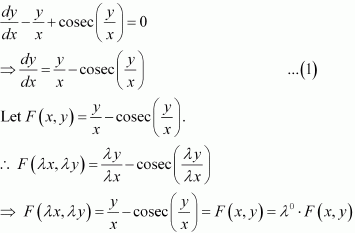Therefore, the given differential equation is a homogeneous equation.

To solve it, we make the substitution as:

y = vx

This is the required solution of the given differential equation.

Question15.

Solution :

Therefore, the given differential equation is a homogeneous equation.

To solve it, we make the substitution as:

y = vx

Question16. A homogeneous differential equation of the formcan be solved by making the substitution:

(A) y = vx

(B) v = yx

(C) vy

(D) x = v

Solution :
We know that a homogeneous differential equation of the formcan be solved by the substitution x = vy

Therefore, option (C) is correct.

Question17. Which of the following is a homogeneous differential equation:

Solution :
Out of the given four options, option (D) is the only option in which all coefficients of x and y are of same degree i.e., 2. It may be noted that yis a term of second degree.

Hence differential equation in option (D) is Homogeneous differential equation.

### Solve The Following Questions.

In each of the following differential equations given in each Questions 1 to 4, find the general solution:

Question1.

Solution :
Given: Differential equation

This is the required general solution of the given differential equation.

Question2.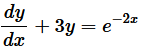Solution :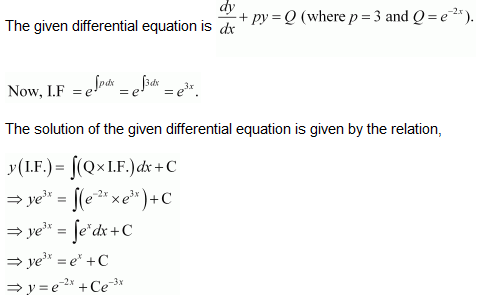Question3.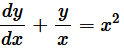Solution :
Given: Differential equationQuestion4.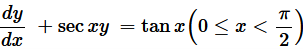Solution :
Given: Differential equationFor each of the following differential equations given in Question 5 to 8, find the general solution:

Question5.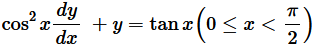Solution :
The given differential equation is:

Question6.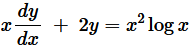Solution :
Given: Differential equationQuestion7.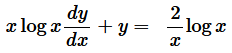Solution :
Given: Differential equationQuestion8.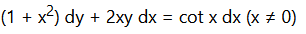Solution :
Given: Differential equationFor each of the following differential equations given in Question 9 to 12, find the general solution:

Question9.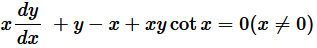Solution :
Given: Differential equationQuestion10.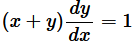Solution :
Given: Differential equationQuestion11.

Solution :
Given: Differential equation

Question12.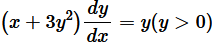Solution :
Given: Differential equationFor each of the differential equations given in Questions 13 to 15, find a particular solution satisfying the given condition:

Question13.

Solution :
Given: Differential equation

This is a linear equation of the form:

Hence, the required solution of the given differential equation is y = cos x – 2 cos2 x.

Question14.

Solution :
Given: Differential equation

This is the required general solution of the given differential equation.

Question15.

Solution :
Given: Differential equation

This is the required particular solution of the given differential equation.

Question16. Find the equation of the curve passing through the origin, given that the slope of the tangent to the curve at any point (xy) is equal to the sum of coordinates of that point.

Solution :

Let F (xy) be the curve passing through the origin.

At point (xy), the slope of the curve will be dy/dx.

According to the given information:

The curve passes through the origin.

Therefore, equation (2) becomes:

1 = C

⇒ C = 1

Substituting C = 1 in equation (2), we get:

x + y + 1 = ex

Hence, the required equation of curve passing through the origin is x + y + 1 = ex.

Question17. Find the equation of the curve passing through the point (0, 2) given that the sum of the coordinates of any point on the curve exceeds the magnitude of the slope of the tangents to the curve at that point by 5.

Solution :

Let F (xy) be the curve and let (xy) be a point on the curve. The slope of the tangent to the curve at (xy) is dy/dx.

According to the given information:

The curve passes through point (0, 2).

Therefore, equation (2) becomes:

0 + 2 – 4 = Ce0

⇒ – 2 = C

⇒ C = – 2

Substituting C = –2 in equation (2), we get:

x + y -4 = – 2ex

⇒y = 4 – x – 2ex

This is the required equation of the curve.

The integrating factor of the differential equation is:

(A) ex

(B) ey

(C) 1/x

(D) x

Solution :

Given: Differential equation

Therefore, option (C) is correct.

The integrating factor of the differential equation

Solution :
Given: Differential equation

Therefore, option (D) is correct.

### Solve The Following Questions.

Question1. For each of the differential equations given below, indicate its order and degree (if defined):

(i)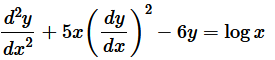(ii)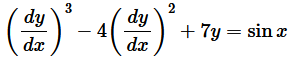(iii)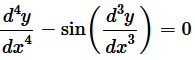Solution :
(i) Given: Differential equationThe highest order derivative present in this differential equation is d2y/dx2 and hence order of this differential equation if 2.

The given differential equation is a polynomial equation in derivatives and highest power of the highest order derivative d2y/dx2 is 1.

Therefore, Order = 2, Degree = 1

(ii) Given: Differential equationThe highest order derivative present in this differential equation is dy/dx  and hence order of this differential equation if 1.

The given differential equation is a polynomial equation in derivatives and highest power of the highest order derivative dy/dx  is 3.

Therefore, Order = 1, Degree = 3

(iii) Given: Differential equationThe highest order derivative present in this differential equation is d4y/dx4 and hence order of this differential equation if 4.

The given differential equation is not a polynomial equation in derivatives therefore, degree of this differential equation is not defined.

Therefore, Order = 4, Degree not defined

Question 2. For each of the exercises given below verify that the given function (implicit or explicit) is a solution of the corresponding differential equation:

Solution :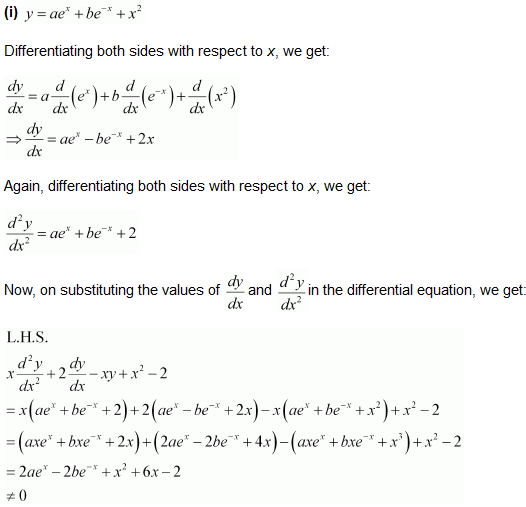Therefore, Function given by eq. (i) is a solution of D.E. (ii).

Therefore, Function given by eq. (i) is a solution of D.E. (ii).

Therefore, Function given by eq. (i) is a solution of D.E. (ii).

Therefore, Function given by eq. (i) is a solution of D.E. (ii).

Question 3. Form the differential equation representing the family of curves where a ia an arbitrary constant.

Solution :
Equation of the given family of curves is

Question 4. Prove that is the general equation of the differential equation  where c is a parameter.

Solution :

Integrating both sides, we get:

Substituting the values of I1 and I2 in equation (3), we get:

Hence, the given result is proved.

Question 5. For the differential equation of the family of the circles in the first quadrant which touch the coordinate axes.

Solution :

The equation of a circle in the first quadrant with centre (aa) and radius (a) which touches the coordinate axes is:

Question 6. Find the general solution of the differential equation

Solution :
Given: Differential Equation

Question 7. Show that the general solution of the differential equationis given by (x + + 1) = (1 – – y – 2xy), where A is parameter

Solution :
Given: Differential equation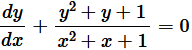Integrating both sides,

Question 8. Find the equation of the curve passing through the point (0,π/4), whose differential equation is sin x cos y dx + cos x sin y dy = 0.

Solution :

The differential equation of the given curve is:

Question 9.Find the particular solution of the differential equation (1 + e2x ) dy + (1 + y2 ) ex dx = 0, given that y = 1 when x = 0.

Solution :

This is the required particular solution of the given differential equation.

Question 10. Solve the differential equation:

Solution :

Question 11. Find a particular solution of the differential equation (x – y) (dx + dy) = dx – dy, given that y = –1, when x = 0. (Hint: put x – y = t)

Solution :

Now, y = –1 at = 0.

Therefore, equation (3) becomes:

log 1 = 0 – 1 + C

⇒ C = 1

Substituting C = 1 in equation (3) we get:

log| x- y| = x + y + 1

This is the required particular solution of the given differential equation.

Question 12. Solve the differential equation:

Solution :

Question 13. Find the particular solution of the differential equationgiven that y = 0 when x = π/2

Solution :

The given differential equation is:

This is the required particular solution of the given differential equation.

Question 14. Find the particular solution of the differential equationgiven that y = 0 when x = 0

Solution :

Now, at x = 0 and y = 0, equation (2) becomes:

This is the required particular solution of the given differential equation.

Question 15. The population of a village increases continuously at the rate proportional to the number of its inhabitants present at any time. If the population of the village was 20,000 in 1999 and 25,000 in the year 2004, what will be the population of the village in 2009?

Solution :

Let the population at any instant (t) be y.

It is given that the rate of increase of population is proportional to the number of inhabitants at any instant.

Integrating both sides, we get:

log kt + C … (1)

In the year 1999, t = 0 and y = 20000.

Therefore, we get:

log 20000 = C … (2)

In the year 2004, t = 5 and = 25000.

Therefore, we get:

In the year 2009, t = 10 years.

Now, on substituting the values of tk, and C in equation (1), we get:

Hence, the population of the village in 2009 will be 31250.

Question 16. The general solution of the differential equation is:

(A)xy = C

(B)= Cy2

(C)= Cx

(D)y = Cx2

Solution :

The given differential equation is:

Therefore, option (C) is correct.

Question 17. The general equation of a differential equation of the typeis:

Solution :

The integrating factor of the given differential equation

The general solution of the differential equation is given by,

Hence, the correct answer is C

Question 18. The general solution of the differential equation  is:

(A) xey + x2 = C

(B) xey + y2 = C

(C) yex + x2 = C

(D) yey x2 = C

Solution :

The given differential equation is:

Therefore, option (C) is correct.

Get 30% off your first purchase!

X
error: Content is protected !!
Scroll to Top